Transcripted Summary

In this section, let's talk about operators.

Operators are the symbols used within conditions. There's relational operators and logical operators.

### # Relational Operators

We've used a few relational operators already, so let's make sure we touch on all of them.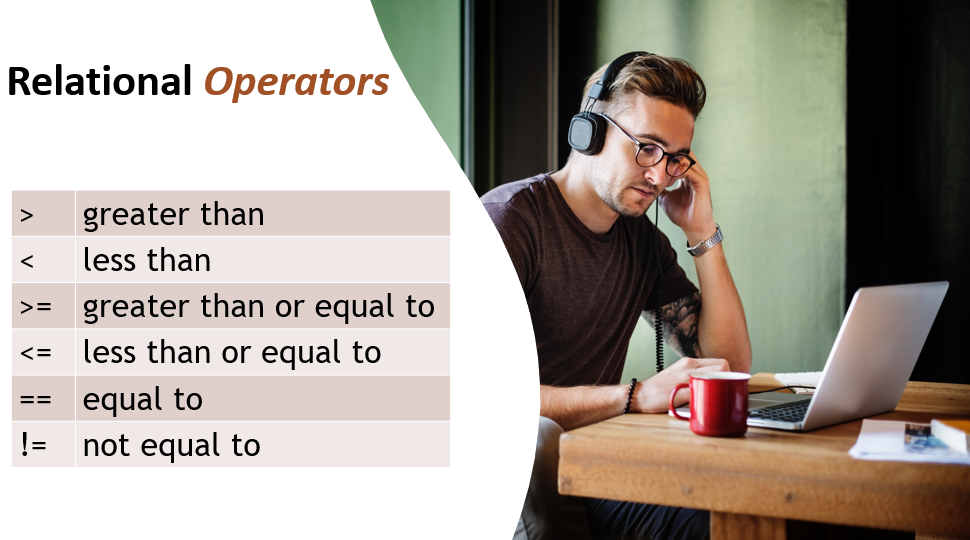We have:

• greater than: `>`
• less than: `<`
• greater than or equal to: `>=`
• less than or equal to: `<=`
• equal to: `==`
• not equal to: `!=`

Let's look at some examples of these operations within boolean expressions.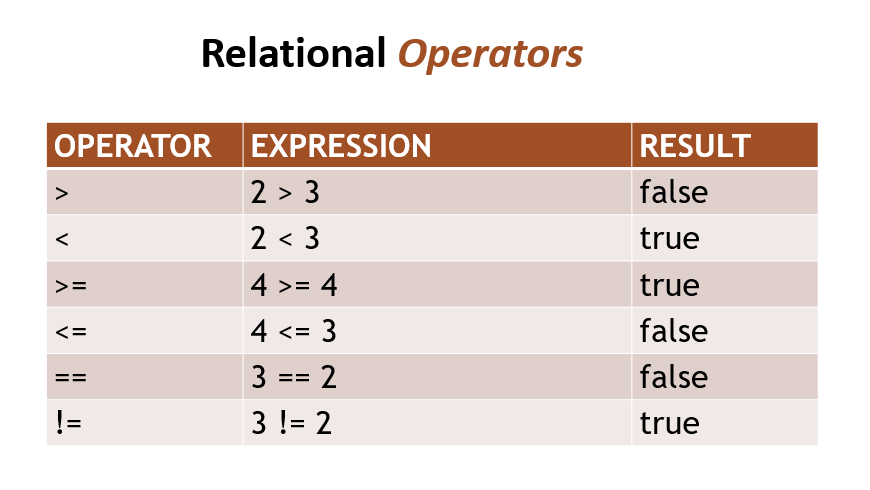Equal Operator: ==

Notice the equal to operator is two equal signs ( == ). In Java the single equal sign ( = ) is used for assignment, like when we assign a value to a variable. So, you cannot use that in a condition to compare values.

If you use the single equal sign when you're supposed to use the double one, awful consequences can occur. Such as this.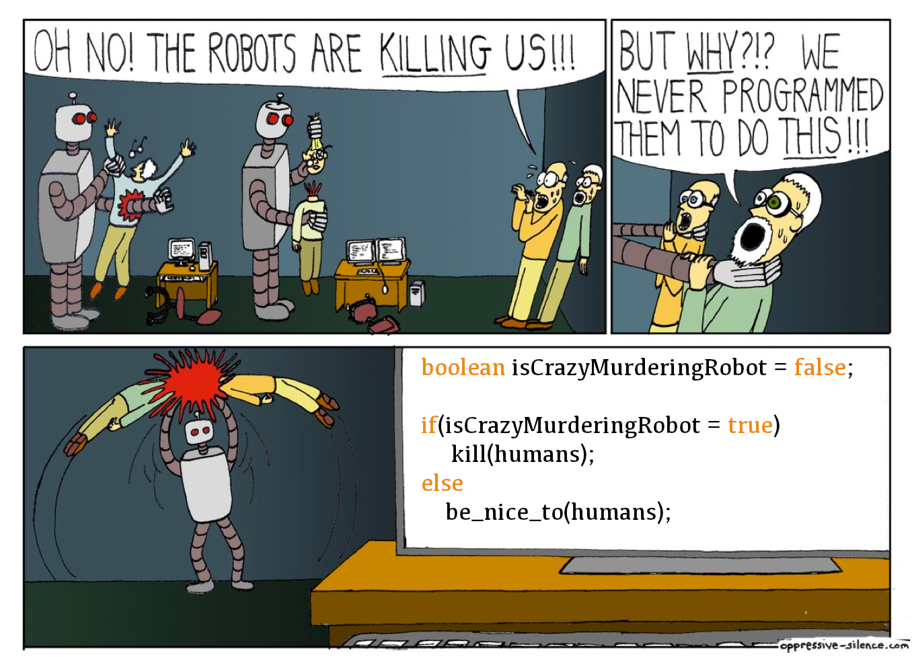Oh no! The robots are killing us. But why? We never programmed them to do this.

Well, let's look at the program. We initialized the “isCrazyMurderingRobot” to false. Then we had a condition to check its value. Only if it's true, do the robots kill the humans. Well, if you look at that condition, it's using the single equal sign which is for assignment. So, what's happening there is it's actually updating the “isCrazyMurderingRobot” value to true. It's not doing a comparison.

So now, you've set isCrazyMurderingRobot to true and we're all dead.

### # Comparing Strings

There will be cases where you want to compare Strings.

You may think to use the == or != for this, however that doesn't necessarily do what you think it does. These two operators will compare the memory locations of both of the Strings, not the values themselves.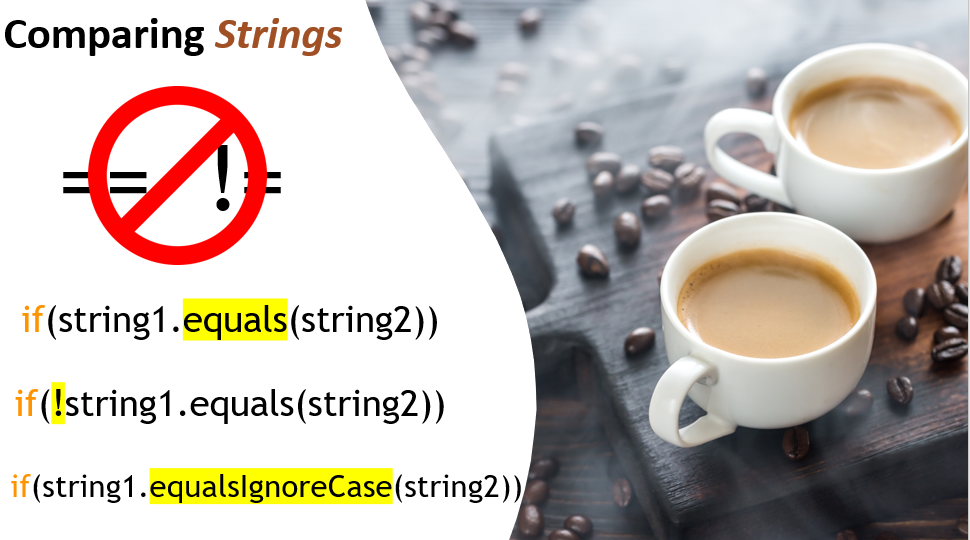If you want to check to see if a String’s value is equal to another String’s value, you need to use the `equals` method of the String.

To check if two String values are not equal, you add the not symbol (!) at the beginning of the condition.

If you don't care about the case of the Strings, then you can use the `equalsIgnoreCase`.

### # Logical Operators

What we saw were relational operators. Now let's talk about logical operators. Logical operators are used to combine two separate conditions in order to get one resulting boolean value.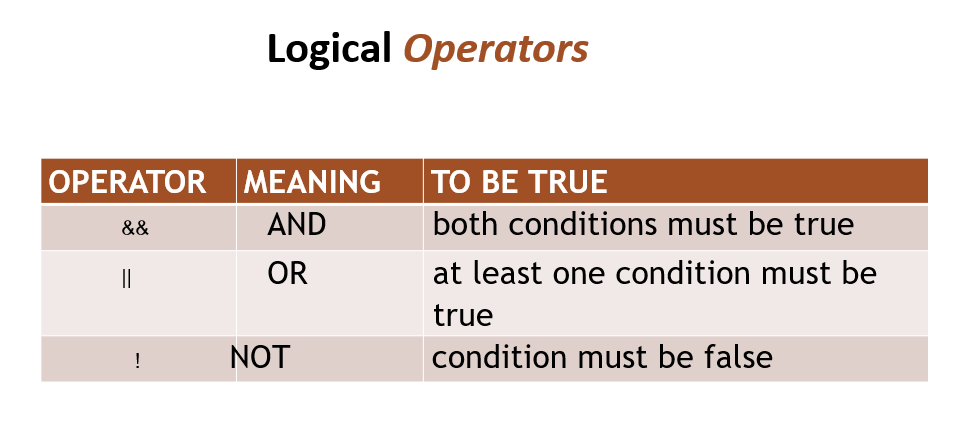Let's look at the first one. It's two ampersands `&&` and this means “AND”. In order for this boolean value to result to true, both of the conditions must be true.

Next, we have the “OR” operator `||`. When this combines two conditions, at least one of those conditions must be true in order for the entire condition to be true.

Then finally the “NOT” operator `!`. The condition itself must be false in order for the resulting condition to be true.

Let's look at a couple examples.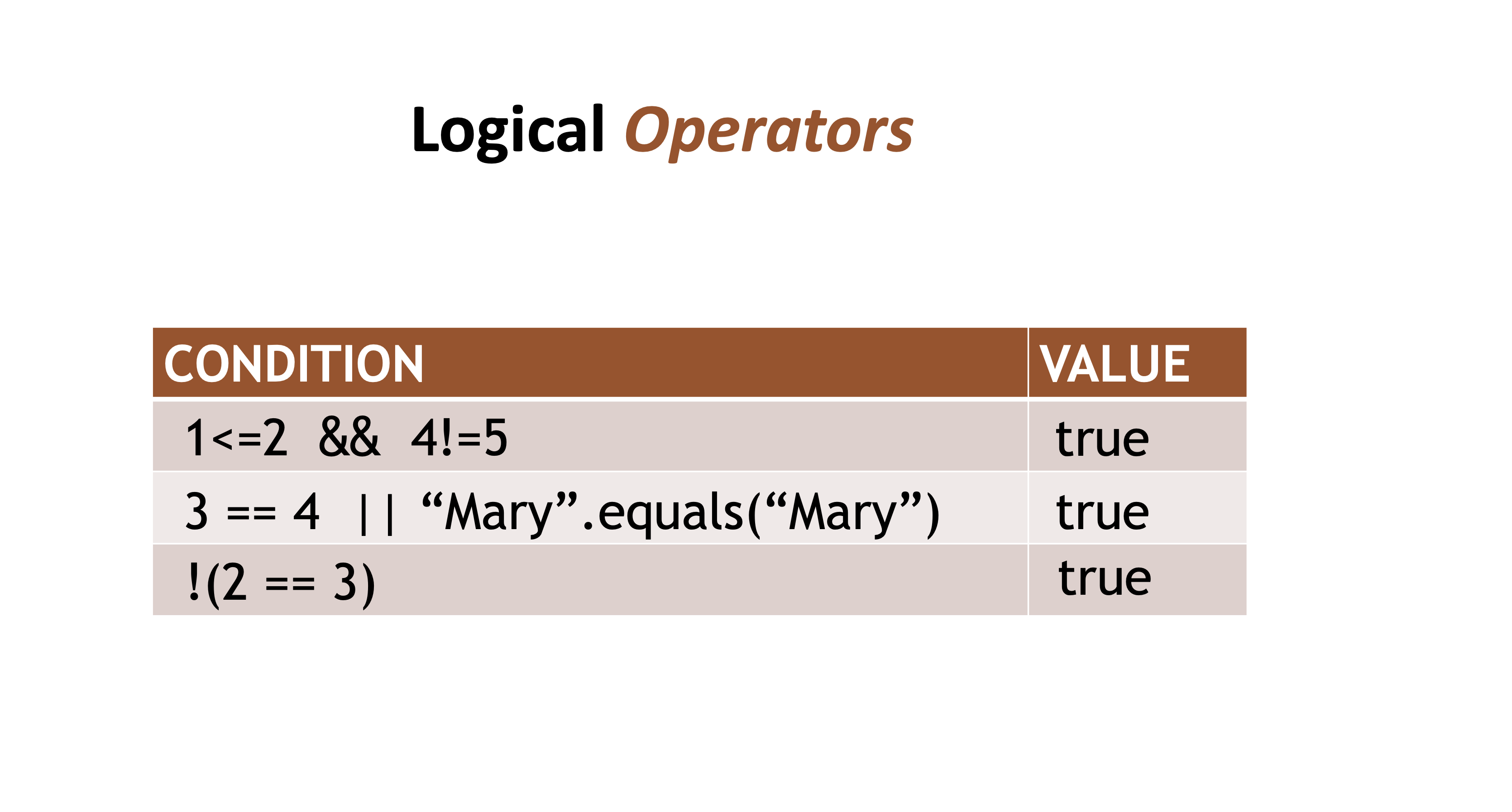Let’s look at the AND

So here we have two conditions: `1<=2 && 4!=5`

The first condition is `1<=2`. By itself, that's a boolean expression and results to true. Now we have the AND operator `&&` which means both of these conditions must be true. So, we look at the second condition: `4!=5`, which is also true. So, the resulting value will be true.

Let's look at the OR

Again, we have two conditions: `3 == 4 || “Mary”.equals(“Mary”)`

The first condition, `3 == 4` is false. But then the next condition, `“Mary”.equals(“Mary”)` is true. With the OR operator `||` only one of the conditions must be true in order for the entire value to result to true.

Then the final one, the NOT

This operator takes the opposite value of the condition. We have `!(2 == 3)`. Well, `2 == 3` is false. but then the NOT operator negates this. So, the value is false, the negation of that is true.

Short Circuit Logic

Now let's look at short circuit logic within these conditions. The AND and OR operators are used to combine two conditions into one.

For the AND operators, both conditions must be true. So, if the very first condition of an AND statement is false, it does not look at the second condition. There's no need to because both would need to be true in order for it to be true. It will look at the first one, if it's false the entire thing will be false. So, this saves a little bit of computational time.

Likewise, with the OR operator, if the first condition is true then it doesn't bother to look at the second condition.

Logical operators can simplify your program by eliminating the need for nested ifs, in some cases.

Let's revisit that Loan Qualifier program, we did and solve it using logical operators instead of the nested ifs.Let's take our LoanQualifier and copy, and then we're going to paste it back into chapter3, then change the class name to `LogicalOperatorLoanQualifier`.

It's also going to change the name of the class itself because the class name must equal the name of the file.

So, we'll change this from “NESTED IFS” to “LOGICAL OPERATORS”.

``````/*
* LOGICAL OPERATORS:
* To qualify for a loan, a person must make at least \$30,000
* and have been working at their current job for at least 2 years.
*/

public class LogicalOperatorLoanQualifier {
``````

Let's go right into this decision logic.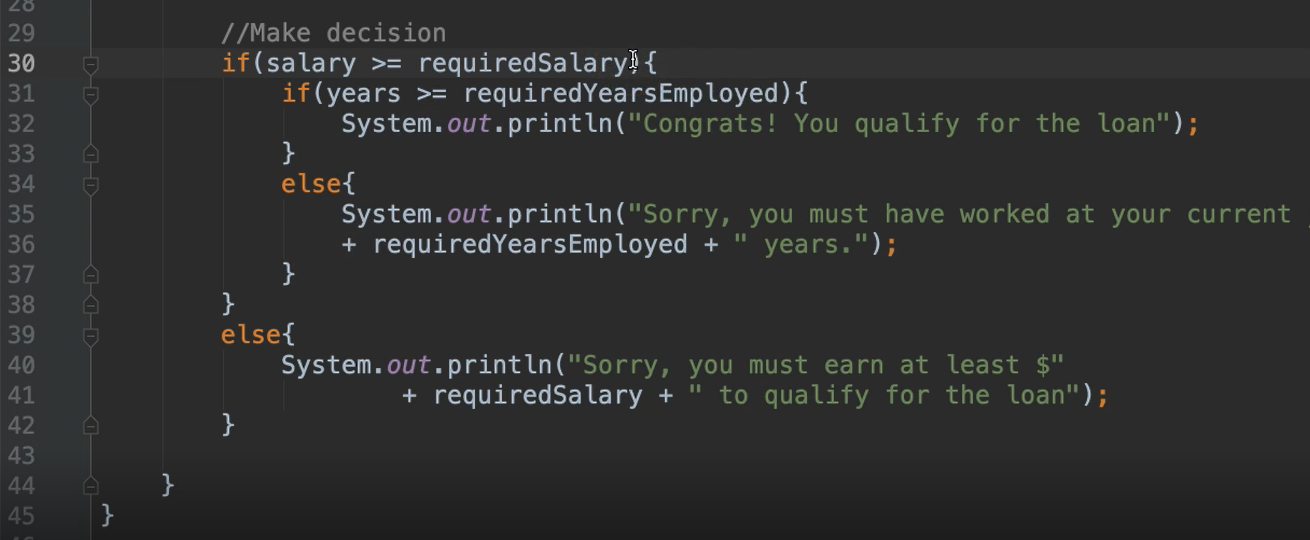So, this is where we have the nested ifs. We can change this.

Instead of using the nested ifs, we can simply use the && operator because both of these conditions must be true in order for them to qualify.

We'll move this to the same line:

``````//Make decision
if(salary >= requiredSalary && years >= requiredYearsEmployed){
System.out.println("Congrats! You qualify for the loan");
}
else{
System.out.println("Sorry, you must earn at least \$"
+ requiredSalary + " to qualify for the loan");
}
``````

Now this `else` statement is no longer applicable, so we can delete it.

Notice with the `else` statement, it showed granular details, so it knew which condition was false. You have no idea which one of these was false. In fact, you won't even go inside of here if either of them is false.

That's one disadvantage. However, this is much cleaner to read. So, you use whichever one that makes sense for your context.

Whew! So that was the decision structures. I know this was a long chapter, but we made it.

I do have a homework assignment for you, if you'd like to do this on your own. If not, that's okay, it's totally optional.

## Optional Independent Exercise

You're going to make a game — the objective of the game is to enter enough change to equal exactly one dollar.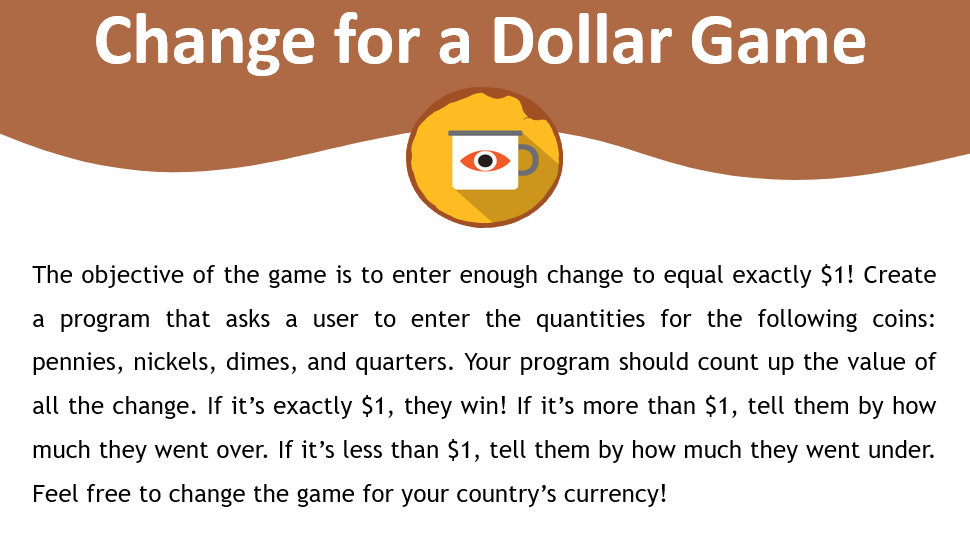So, you're going to create a program that asks the user to enter the quantities for the following coins.

• You'll ask them how many pennies would you like?
• How many nickels would you like?
• How many dimes?
• And then, how many quarters?

What your program is going to do is count up the value of all of the change that they answered.

• If it's exactly one dollar then they win the game.
• If it's more than one dollar, then you need to tell them that it's more than one dollar and how much they went over.
• If it's less than one dollar, tell them how much they went under.

Feel free to change this game for your country's currency, so if you don't use dollars and you use something else that's fine.

So, for an example of this let's say that I ask the user:

• How many pennies? - And they said 0.
• How many nickels? - 0
• How many dimes? - 0
• How many quarters? - 4

Then my program would know that, okay quarters are worth 25 cents. There's 4 of these, let me multiply those and get the value. Oh! that equals one dollar, so I let them know that they win. So that's an example of the game.

Good luck!

Solution

Programming can be done many different ways, but here’s my solution.